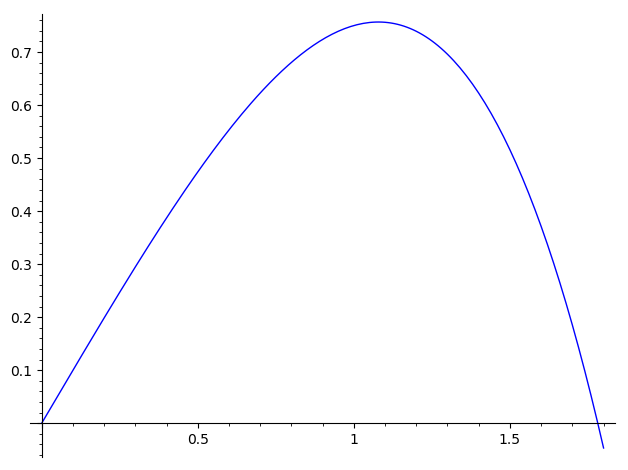Ask Your Question

# Solving 2nd order ode with desolve_system_rk4

Dear All,

I have the following nonlinear ode to solve numerically:

$$\ddot q(t) = -\dot q(t)^2 - q(t)$$.

Since desolve_system_rk4 cannot solve 2nd order ode I make this ode a system of two 1st order odes in the following way:

$$q'(t) = qdot(t)$$ $$qdot'(t) = -qdot(t)^2 - q(t)$$

However desolve_system_rk4 cannot still solve this 2 odes numerically. It raises an error. Any help is appreciated.

Here is the code I wrote:

t = var('t')
q = function('q')(t)
qdot = function('qdot')(t)
# Equations of motion
eom1 = q.diff(t) == qdot(t)
eom2 = qdot.diff(t) == -(qdot(t))^2 - q(t)
# Initial conditions
q0 = 0
qdot0 = 1
sol = desolve_system_rk4([eom1, eom2], [q, qdot], ivar = t , ics = [q0, qdot0])

edit retag close merge delete

## 1 Answer

Sort by » oldest newest most voted

Hello, @F. Semih Dündar! I can see a couple of problems with your code. First of all, there is no need to define $q$ and $qdot$ as formal functions, since that is implicitly assumed. Second, you only need to pass the RHS (right hand side) of the differential equations. Finally, the ics parameter must be of the form [t0, q0, qdot0].

With those considerations, your code should look like this:

var('t, qdot, q') # independent variable and functions
# Equations of motion
eom1 =  qdot
eom2 = -(qdot(t))^2 - q(t)
# Initial conditions
q0 = 0
qdot0 = 1
sol = desolve_system_rk4([eom1, eom2], ivar=t, vars=[q, qdot], ics = [0, q0, qdot0], end_points=1.8, step=0.01)
S = [(t, q) for t, q, qdot in sol]
list_plot(S, plotjoined=True)


I think a few additional clarifications are in order. As mentioned, you only need the RHS of the DEs; that is why I removed part of $oem1$ and $oem2$ (specifically, the LHS and the ==). The vars parameter implicitly indicate that your system is of the form

$$q' = oem1$$ $$qdot' = oem2$$

so this code defines the same system you intended.

I have used the end_points=1.8 parameter in order to solve in the interval $[0, 1.8]$---after $t\approx1.8$, the value of $q$ is negative, which I assumed not to be desirable, although I don't know the context in which you are trying to solve this (so maybe you would like to modify that parameter if needed).

On the other hand, when I plotted the solution, due to the small domain, I obtained a pointy curve, so I used the step=0.01 parameter, in order to decrease the step length, and obtain a more refined solution.

The last two lines are intended to obtain a plot of $q$ alone, without including $qdot$. (I assume they are clear to you, but feel free to ask otherwise.)

Here is the resulting image:I hope this helps!

more

## Comments

Why is "qdot" in the equation eom1? Maybe it should be like F.SemihDündar wrote, i.e. eom1 = qdot (t). In this case, we will receive another solution.

Hello, @aszanti! That is not correct. Observe the order in which the first and third arguments for desolve_system_rk4 are given: the first one is [oem1, oem2], and the second (vars) is [q, qdot]. As I mentioned in my answer, the syntax of the command then implies that

$$q'=oem1=qdot$$ $$qdot′(t)=oem2=−qdot(t)2−q(t)$$

which is exactly the system that @F. Semih Dündar wants to solve. In particular, sol will have the form

$$sol = [t, q(t), qdot(t)],$$

for $t\in[0,1.8]$.

Remember, $oem1$ is not an equation, but the the RHS of an equation. The LHS is implicitly equal to the derivative of the first argument of the vars parameter.

Hello dsejas! I understand now, thank you very much for this explanation.

## Your Answer

Please start posting anonymously - your entry will be published after you log in or create a new account.

Add Answer

## Stats

Asked: 2020-06-01 10:58:23 +0200

Seen: 121 times

Last updated: Jun 02 '20